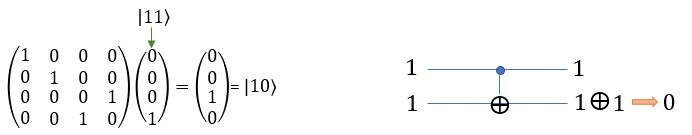Quantum Computing Quamtum Gate - CNOT (Controlled NOT)   As the name of the gate implies, CNOT gate is a type of NOT gate but not exactly same as 'NOT' gate. Confusing ? -:) Yes, It is confusing. CNOT is a type of NOT gate which performs the NOT operation only under a specific condition. If the specific condition is not met, it does not perform any operation and just pass through the input Qbit without any modification. This gate has two input pins and two output pins. The first pin is called 'control' pin that determines whether the gate should perform 'NOT' operation or not.   If you take a look at the symbol of CNOT gate, you would notice that this gate has two input and two output. The first line (the upper input pin) represents the one that specifies the condition and the second line (the lower input pin) indicates the bit that is the target for NOT operation. (NOTE : this gate opearate on two Qbits but the dimension of matrix is 4x4. If this make sense to you, it mean that you have pretty good understanding of matrix/vector representation of Qbit operation. Otherwise, try reading Qbit page)We can represent this in a little different way as shown below. x indicate the control Qbit and y is input Qbit. As you see, on the upper line the Qbit x (the control) goes through without any changes. But on the lower line the Qbit y is XORed with the condition bit x.  The XOR mentioned here is XOR operation in classical bit operation.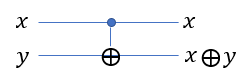If you are familiar with how XOR operation works and give some time think of the meaning of the above operation, you would understand how CNOT gate works...  if this is not yet clear, let's look at some specific examples as below.   Simply put, if x is 0, output (x XOR y) is same as y (meaning no NOTing). if x is 1, the output (x XOR y) is same as [NOT y].     As the first example, let's take the case where the two Qbit |00> goes through CNOT gate. In this case, the conditional Qbit is |0> and input Qbit is |0>.  Since the conditional Qbit is |0>, the NOT operation is not performed for the input Qbit. So the result is |0>.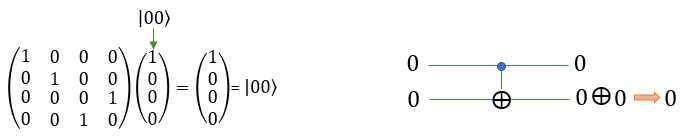As the first example, let's take the case where the two Qbit |01> goes through CNOT gate. In this case, the conditional Qbit is |0> and input Qbit is |1>.  Since the conditional Qbit is |1>, the NOT operation is not performed for the input Qbit. So the result is |1> .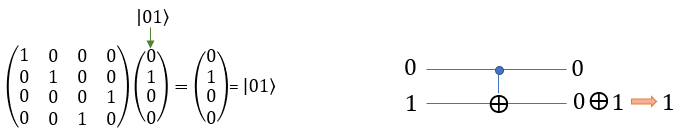As the first example, let's take the case where the two Qbit |10> goes through CNOT gate. In this case, the conditional Qbit is |1> and input Qbit is |0>.  Since the conditional Qbit is |1>, the NOT operation is performed for the input Qbit. So the result is |1> (i.e, NOT |0> = |1>)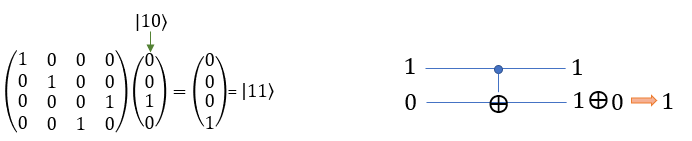As the first example, let's take the case where the two Qbit |10> goes through CNOT gate. In this case, the conditional Qbit is |1> and input Qbit is |1>.  Since the conditional Qbit is |1>, the NOT operation is performed for the input Qbit. So the result is |0> (i.e, NOT |1> = |0>)